# Interferometry Tutorial

The page is created to provide a short overlook on the interferometry theory and techniques.

## Monochromatic source

Let's consider a monochromatic point source located at infinity and two telescopes that are observing the source in the direction S. The telescopes are on a reference axis, with each an x-coordinate x1 and x2 (cf fig.1).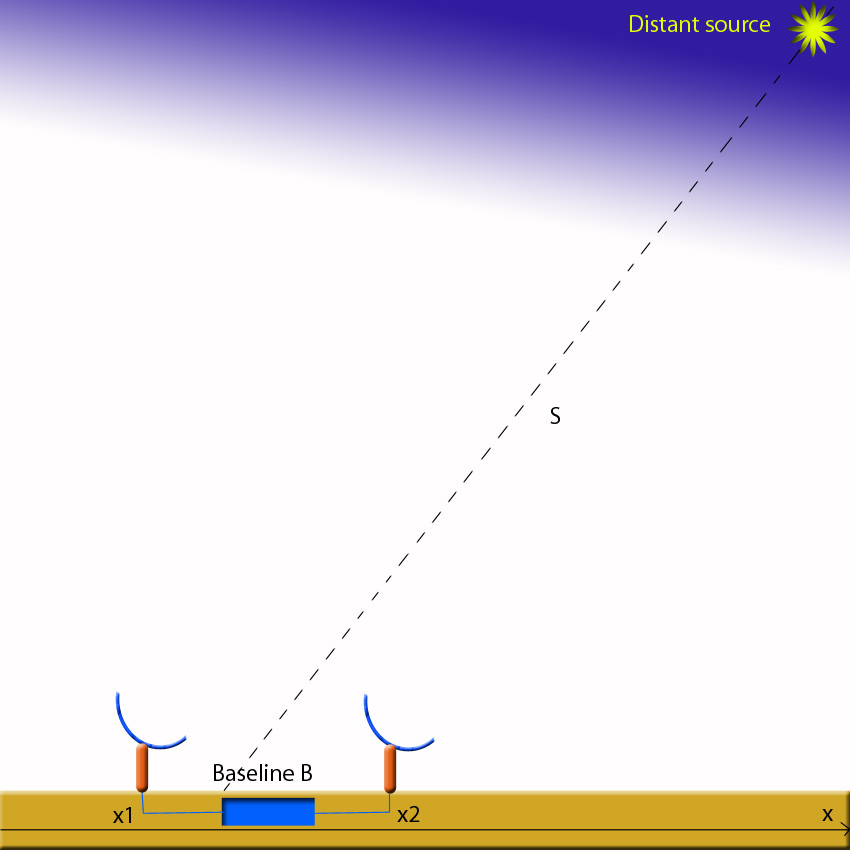Fig. 1: Observation of a source S by two telescopes

They are also separated by a baseline B given by: B=x2-x1 . The source being very far, the wavefronts can be considered as plane. The waves observed by the telescopes are given by:

Φ1 ~ exp(-ik*x1.s)exp(-iωt)

Φ2~ exp(-ik*x2.s)exp(-iωt)

For x1= 0, the expressions become:

Φ1~ exp(-iωt)

Φ2 ~ exp(-ik*B.s)exp(-iωt)

N.B: The negative sign of the first exponential of Φ2 is due to the fact that the plan wavefront arrives later on the telescope 1.

The interferometer is equipped with delay lines that offer the possibility to add a supplementary OPD. If we consider that the first delay line adds the OPD1=d1 to the travel of the beam observed by the first telescope and OPD2 = d2 added by the other delay line, the expressions become:

Φ1~ exp(ik*d1)exp(-iωt)

Φ2~ exp(ik*d2)exp(-ik*B.s)exp(-iωt)

The beams are then combined by a beam combiner. The resulting interferences have the following form:

Φ = Φ12~ exp(-iωt)[exp(ikd1)+exp(ikd2)exp(-ik*B.s)]

The resulting intensity is not merely the simple addition of the intensities 1 and 2 but is modulated by the phenomenon of interference:

Imoy ~ Φ*Φ conj = 2(1+cos(k*[s.B+d1-d2]))

Typically we have the general form:

Imoy = 2I0(1+cos(k*D))

that gives the traditional fringe scheme: I(D) (shown in fig.2), with a sinusoidal form, varying between 0 and 2I0, with I0proportional to A* F (and not equal because of some loss in the VLTI optical train concerning F) where A is the collecting area of the apertures and F the incident source flux power in units of incident energy per units time per units cross-sectional area. Two peaks are separated by: Δs=λ/b.Fig. 2: Theoretical fringes ( I / (2I0) = f(D) )

## Polychromatic source

The interferometers have a limited bandwidth Δλ, have a transmissivity depending on the frequency given by η(ν):F(ν)=F*ην*dν . Then the brightness has the following form:Concerning the coherence, it can be divided in two aspects, the spatial and the temporal coherence. With astronomical sources, the spatial coherence is always verified because the star is very far from the telescopes, thus the beams that interfere have a null phase difference. On the other side the temporal incoherence comes from the fact that a source is never monochromatic: always a spectral widthΔλ centered on λ0, the interferences will appear under the condition: λ02/Δλ = λcoh. λcohbeing the coherence length

By integrating the general equation above, we get: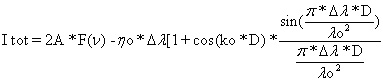That gives the previous fringe pattern (monochromatic case)modulation with an envelope function. This fringe pattern is characteristic of a polychromatic source (cf fig.3). The envelope function can be written as: 1+M(λcoh,D)cos(k0*D) where M is the modulation of the envelope (its value is given by the Fourier transform of the system band pass).Fig. 3: The fringe pattern of a polychromatic source

## Phase reference for interferences

In general a source point is given this equation for the brightness modulated by: k0*D=k0*(s0.B+d1-d2).

If we define d2-d1=s0.B, we know that we will be at maximum of the fringes envelope function for a source at location s0.

Now we can see what would be the response for s=s0 + Δs :

I = 2*A*F(1+M(λcoh,ΔD)cos(k0(s.B-s0.B)))

i.e. I = 2*A*F(1+M(λcoh,ΔD)cos(k0*ΔD))

where ΔD = Δs.B. In this construction the sky position s0 (reference) as defined by the relative delay d2-d1 is the phase reference of the interferences fringes on the sky.

## Extended sources

Let's describe the source intensity spatial distribution with the function F(s) in units of power incident per area per solide angle on the sky. λ being the wavelength .

The efficiency of the telescope is a function of the sky position and we will use A(s,s0).The extended source, the star, can be decomposed in an incoherent sumof infinitesimal source points, the brightness can so be written like:Let's simplify by returning to a monochromatic source (a givenλ). The optical interferometers are measuring fringes byintroducing a small added phase in one of the two delay lines δ :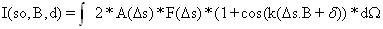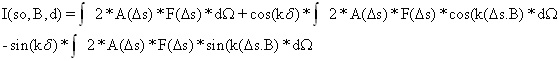Introducing the complex visibility: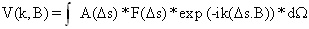leads to the following form for the brightness:

I(s0,B,δ) = 2I0 + 2Re{V*exp(ikδ)}

where 2I0 is the maximum brightness of the two apertures.

In the case of PRIMA the sources are a bright guide star and a fainter one, far from the telescopes, separated by a narrow angle.Let's assume that s0 has the following coordinates (0,0,1) and Δs perpendicular to s0: (α,β,0). Then the complex visibility takes the form:It is further conventionnal to introduce spatial frequencies u = Bx/λ and v = By/λ , giving the final form of the complex visibility: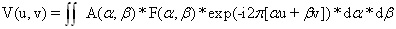that is complex and has the dimension of a power.

## Image synthesis by discrete visibility measurements

The precedent form of the complex visibility is a two-dimensional fourier transform of the distribution of the brightness F. Thus the visibility (provided we can measure it) can be used to compute the brightness distribution of the observed object:Thus in order to get an image, a set of visibility measurements in the (u,v) plane needs to be collected before defining F. In practice aserie of baselines Bi tracking the same object provide a set of discrete visibility measurements Vi(ui,vi). The information is collected in (u,v)-maps.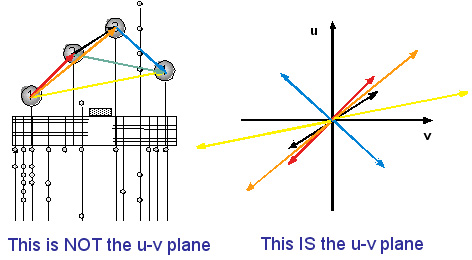Fig. 4: Set of baselines to realize a (u,v)-mapFig. 5: (u,v)-coverage with a UT

The accuracy of the image synthesis will of course depend on the coverage of the (u,v)-plane (cf fig.5).

But to reconstruct the brightness distribution a sampling function is defined to take into account the measurements:

S(u,v) = Σδ(u-ui)*δ(v-vi)

leading to a new expression for F :

Fd =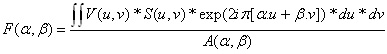Fd refers to the "dirty"brightness distribution that is related to the true brightness distribution by a convolution with an effective point-spread function(PSF) or synthesized beam ("dirty beam"):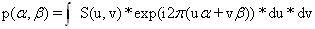Using the convolution theorem:

Fd(α,β) = F(α,β) * p(α,β)

the true brightness distribution can be obtained from the deconvolution of this product. This allows toreconstruct the image (cf. fig.6).Fig. 6: Reconstructed image

N.B: If the object is not symmetrical (as a spiral nebula for example), its fourier transform is complex instead of being purely real.

## Contrast and physical interpretations

The definition given for the complex visibility gives it a dimension of power. It's amplitude measures the difference of intensity between the fringes (V is capturing the coherent response of the interferometer to the astronomical source). In reality the optical interferometer measures the normalized fringe power, the fringes power relative to the total power collected from the source, this is quantified as the Michelson fringe visibility :

VM = (Imax-Imin)/(Imax+Imin)

νM has no dimension and varies in the [0,1] interval. The Michelson visibility can be linked to the complex visibility:

VM = V(k,B)/2I0

Thus the following expression is obtained for the brightness: I = 2I0[1+Re{Vexp(ikδ)}]

and VM = abs(V)

## Visibility of common sources morphologies

### Point source

Many astronomical sources are sufficiently distant and isolated tobe considered as point-like. Thus the brightness distribution of a source with the coordinates (α00) relative to the phase reference is:

F0*δ(α-α0)*δ(β-β0)

The total collected power:is given by the complex visibility: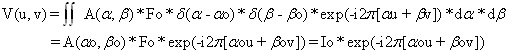implying the following value for the normalized visibility: ν = exp(-i2[πα0u+β0v]) (a pure phase).

The interferometer detected power becomes:or more simply:

I = 2I0(1+cos(k(Δs.B)))

where we have identified α00to Δs and set δ = 0.

### Uniform Disk

With a high angular resolution, as offered by the VLTI, a certain number of sources can be resolved. Some of the most important objects are nearby stars. A reasonable approximation to the brightness distribution of a resolved star is the model of an uniform disk.

In polar coordinates, the model can be written as:

F(ρ) = F0 (ρ<θ/2) ,

where ρ is an angular offset on the celestrial sphere from the center of the source and θ the diameter of the source. Knowing itwe determine the total collected power:

Io = A0*F0*π(θ2/4) (the crossing area of the source is known).

The complex visibility is given by:To compute the visibility for the disk, the two-dimensional Fourier tranform of an axisymmetric function needs to be considered, let's takeƒ=ƒ(ρ) to evaluate: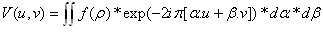with α = ρcosθ and β = ρsinθ

To perform the estimation, it's convenient to take: u = νrcosΦ and v = νrsinΦ and to work with the phases and the amplitudes (νr is the radial spatial frequency). F becomes:F is by construction an axisymmetric function, we can take Φ = 0without losing the generality. The θ-integral can be performedwith:where J0 is the zeroth-order Bessel function. Finally: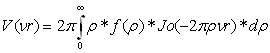Back to the uniform disk, the visibility can be written as: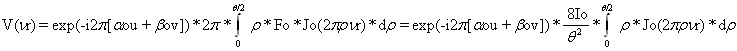N.B:, where Ji (i = 0 to 1) are the Bessel functions.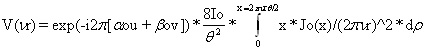Recall νr is a radial spatial frequency: νr2 = u2 + v2 = (B/λ)2, making the visibility:The normalised visibility is then: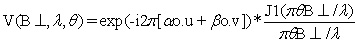Because of the noise properties, interferometers typically measure the squared normalised visibility. It's amplitude is:

ν(B,λ,θ)2 = [2J1(πθB/λ)/(πθB/λ)]2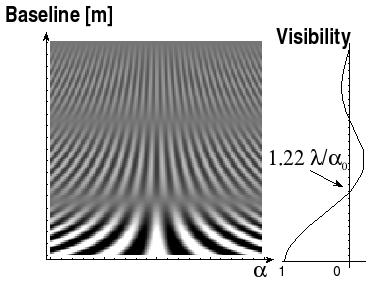Fig. 7: Evolution of fringes with the baseline

### Multiple stellar systems

Many ot the stars observed belong to a multiple star system. To study such systems the response of the interferometer can be considered as a collection of quasi uniform stellar disks. The total received power is the sum of the powers of the visible sources:

P0 = Σ(j) Pj.

As the Fourier transform is linear the complex visibility is the sum of the complex visibilities of the constituent disks af the positions(αjj)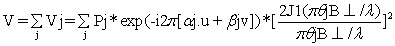The normalised visibility is: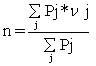e.g. The best known example concerns binaries (cf picture below).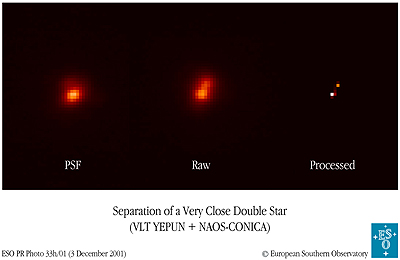The normalised visility is written: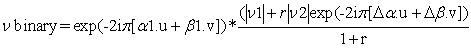with r = I2 / I1, Δα = α2 - α1, Δβ = β2 - β1

when the observable is νbinary2 ,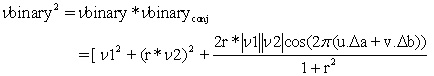with sbinary = (Δα,Δβ)

These corrections are due to finite bandwidth effects when B.sbinaryis more that a few fringe spacings. The exact form of these correctionsdepends on the fringe tracking process. In the limit of a point-like source, the previous expression simplifies to: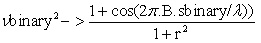When sbinary -> 0 , the two components of the binary system are not resolved by the interferometer. With increasing sbinary, B.sbinary-> λ/4,νbinary2-> 1/2 and the binary system becomes resolved by the interferometer.

Finally, νbinary2 the modulus of the visibility exhibits sinusoidal variations as a function of B.sbinary varying..Fig. 8: Visibility for a binary system